# Graphs of Geometric Solids

Relevant articles: , , , , , , , , , , , .

[hide]

# Graphs of Geometric Solids

Results have been obtained on the 5 Platonic solids, on 12 of the 13 Archimedean solids, and also on the Pseudo-rhombicuboctahedron.

## Spectrum Results

Table 1 summarises the known results on the spectrum problem for the 5 Platonic solids (the tetrahedron, the cube, the octahedron, the dodecahedron and the icosahedron), the 13 Archimedean solids (the cuboctahedron, the truncated tetrahedron, the trucated cube, the truncated octahedron, the rhombicuboctahedron, the truncated cuboctahedron, the icosidodecahedron, the rhombicosidodecahedron, the truncated icosahedron, the truncated dodecahedron, the truncated icosidodecahedron, the snub cube and the snub dodecahedron) and the pseudo-rhombicuboctahedron. An explanation of the sources of most of these results is given in . New results, coming after  was published, and a correction to  are discussed below.

The spectrum problem for the octahedron was solved in 1992 by Griggs et al , with the exception that the case$n=81$ was left unresolved. An octahedron design of order$n=81$ was subsequently constructed by Adams et al in . In , the solution of the spectrum problem for the octahedron was incorrectly attributed to Horak and Rosa .

The spectrum problem for the rhombicuboctahedron was completely solved by Forbes et al .

The spectrum problem for the dodecahedron was completed by Adams, Bryant, Forbes and Griggs .

The spectrum problems for the truncated tetrahedron, the truncated cube and the trucated octahedron were completely solved by Forbes, Griggs and Holroyd .

The spectrum problem for the icosahedron was completed, except that the existence of an icosahedron design of order$n$ is left unresolved for$n\in\{21,141,156,201,261,276\}$, by Forbes and Griggs .

Forbes and Griggs  have shown that if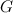$G$ is the graph of the truncated cuboctahedron, the icosidodecahedron, the rhombicosidodecahedron, the truncated icosahedron, the truncated dodecahedron, the truncated icosidodecahedron or the snub cube, then there is a$G$-design of order$n$ for all$n\equiv 1\ ({\rm mod}\ 2e)$ where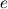$e$ is the number of edges in$G$. Also in , the spectrum problem is completely solved for the graph of the pseudo-rhombicuboctahedron and it is shown that there exists a$G$-design of order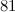$81$ where$G$ is the graph of the icosidodecahedron, and that there exists a$G$-design of order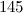$145$ where$G$ is the graph of the truncated dodecahedron.

There are currently no known results on the spectrum problem for the snub dodecahedron.

Designs covered by Wilson’s Theorem  are ignored in the listed possible exceptions in Table 1.

Table 1
 Graph Spectrum Possible exceptions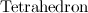${\rm Tetrahedron}$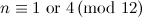$n \equiv 1 {\rm \ or \ } 4 \,({\rm mod \ }12)$$\emptyset$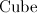${\rm Cube}$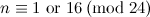$n \equiv 1 {\rm \ or \ } 16 \,({\rm mod \ }24)$$\emptyset$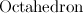${\rm Octahedron}$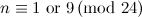$n \equiv 1 {\rm \ or \ } 9 \,({\rm mod \ }24)$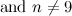${\rm and \ } n\neq 9$$\emptyset$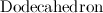${\rm Dodecahedron}$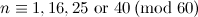$n \equiv 1,16,25 {\rm \ or \ } 40 \,({\rm mod \ }60)$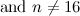${\rm and \ } n\neq 16$$\emptyset$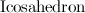${\rm Icosahedron}$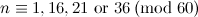$n \equiv 1,16,21 {\rm \ or \ } 36 \,({\rm mod \ }60)$$21$,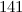$141$,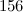$156$,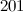$201$,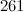$261$ and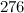$276$${\rm Cuboctahedron}$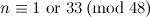$n \equiv 1 {\rm \ or \ } 33 \,({\rm mod \ }48)$$\emptyset$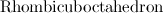${\rm Rhombicuboctahedron}$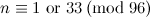$n \equiv 1 {\rm \ or \ } 33 \,({\rm mod \ }96)$$\emptyset$${\rm Truncated\ tetrahedron}$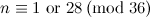$n \equiv 1 {\rm \ or \ } 28 \,({\rm mod \ }36)$$\emptyset$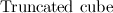${\rm Truncated\ cube}$$n \equiv 1 {\rm \ or \ } 64 \,({\rm mod \ }72)$$\emptyset$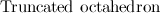${\rm Truncated\ octahedron}$$n \equiv 1 {\rm \ or \ } 64 \,({\rm mod \ }72)$$\emptyset$${\rm Truncated\ cuboctahedron}$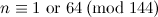$n \equiv 1 {\rm \ or \ } 64 \,({\rm mod \ }144)$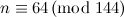$n \equiv 64 \,({\rm mod \ }144)$${\rm Icosidodecahedron}$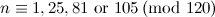$n \equiv 1,25,81 {\rm \ or \ } 105 \,({\rm mod \ }120)$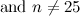${\rm and\ }n\neq 25$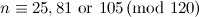$n \equiv 25,81 {\rm \ or \ } 105 \,({\rm mod \ }120)$ with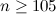$n\geq 105$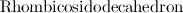${\rm Rhombicosidodecahedron}$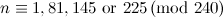$n \equiv 1,81,145 {\rm \ or \ } 225 \,({\rm mod \ }240)$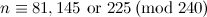$n \equiv 81,145 {\rm \ or \ } 225 \,({\rm mod \ }240)$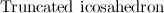${\rm Truncated\ icosahedron}$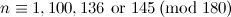$n \equiv 1,100,136 {\rm \ or \ } 145 \,({\rm mod \ }180)$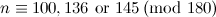$n \equiv 100,136 {\rm \ or \ } 145 \,({\rm mod \ }180)$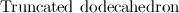${\rm Truncated\ dodecahedron}$$n \equiv 1,100,136 {\rm \ or \ } 145 \,({\rm mod \ }180)$$n \equiv 100,136 {\rm \ or \ } 145 \,({\rm mod \ }180)$ with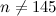$n\neq 145$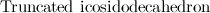${\rm Truncated\ icosidodecahedron}$$n \equiv 1,136,145 {\rm \ or \ } 280 \,({\rm mod \ }360)$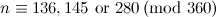$n \equiv 136,145 {\rm \ or \ } 280 \,({\rm mod \ }360)$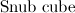${\rm Snub\ cube}$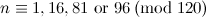$n \equiv 1,16,81 {\rm \ or \ } 96 \,({\rm mod \ }120)$${\rm and\ }n\neq 16$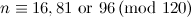$n \equiv 16,81 {\rm \ or \ } 96 \,({\rm mod \ }120)$ with$n\geq 81$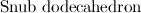${\rm Snub\ dodecahedron}$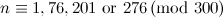$n \equiv 1,76,201 {\rm \ or \ } 276 \,({\rm mod \ }300)$$n \equiv 1,76,201 {\rm \ or \ } 276 \,({\rm mod \ }300)$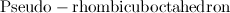${\rm Pseudo-rhombicuboctahedron}$$n \equiv 1 {\rm \ or \ } 33 \,({\rm mod \ }96)$$\emptyset$

## Notes

• The tetrahedron, being the complete graph on four vertices, is covered in the section on complete graphs.
• The cube is covered in section on cubes.

## References

1. 1.0 1.1 Adams, P., Billington, E. J., and Rodger, C. A. Pasch decompositions of lambda-fold triple systems, J. Combin. Math. Combin. Comput. 15, 53–63 (1994).
2. Adams, P. and Bryant, D. E. Decomposing the complete graph into Platonic graphs, Bull. Inst. Combin. Appl. 17, 19–26 (1996).
3. 3.0 3.1 3.2 3.3 3.4 Adams, P., Bryant, D., and Buchanan, M. A survey on the existence of G-designs, J. Combin. Des. 16, 373–410 (2008).
4. 4.0 4.1 Adams, P., Bryant, D., Forbes, A. D., and Griggs, T. S. The completion of the spectrum problem for the Dodecahedron, Preprint,
5. 5.0 5.1 Forbes, A. D., Griggs, T. S., and Holroyd, F. C. Rhombicuboctahedron designs, J. Combin. Math. Combin. Comput. 75, 161 (2010).
6. 6.0 6.1 Forbes, A. D., Griggs, T. S., and Holroyd, F. C. Truncated tetrahedron, octahedron and cube designs, Submitted,
7. 7.0 7.1 Forbes, A. D. and Griggs, T. S. Icosahedron Designs, Preprint,
8. 8.0 8.1 8.2 Forbes, A. D. and Griggs, T. S. Archimedean Graph Designs, Preprint,
9. Grannell, M. J., Griggs, T. S., and Holroyd, F. C. Cuboctahedron designs, J. Combin. Math. Combin. Comput. 35, 185–191 (2000).
10. 10.0 10.1 Griggs, T. S., de Resmini, M. J., and Rosa, A. Decomposing Steiner triple systems into four-line configurations, 52, 215–226 (1992).
11. 11.0 11.1 Horák, P. and Rosa, A. Decomposing Steiner triple systems into small configurations, Ars Combin. 26, 91–105 (1988).
12. 12.0 12.1 Wilson, R. M. Decompositions of complete graphs into subgraphs isomorphic to a given graph, Proceedings of the Fifth British Combinatorial Conference (Univ. Aberdeen, Aberdeen, 1975), Congressus Numerantium, No. XV, Utilitas Math., Winnipeg, Man. 647–659 (1976).